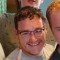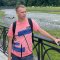# Defining RelationshipsLast updated Dec 21st, 2019 | Page history | Improve this page | Report an issue

We're going to need to define some relationships between our tables so xPDO can communicate properly between them. xPDO deals with two types of relationships, aggregate and composite.

## Aggregate Relationships¶

An aggregate relationship in xPDO is relationship between two tables where the secondary table is an aggregate of the primary table in such a way that if the object in the primary table is deleted, the related object in the secondary table should still exist.

A great example of this is a collection of Crayons in a Box. The relationship from the Crayons to the Box is an aggregate relationship. If you delete a crayon object, it's related box object should not be removed (because it might contain other crayons). Our crayon object would be defined in our XML schema like this:

``````<object class="myCrayon" table="crayons" extends="xPDOSimpleObject">
<field key="box" dbtype="int" precision="10" phptype="integer" null="false" default="" />
<aggregate alias="Box" class="myBox" local="box" foreign="id" cardinality="one" owner="foreign" />
</object>
``````

Note the attributes:

• alias - Relationships in xPDO allow for 'aliases', which can differentiate between two different relationships that refer to the same foreign key.
• class - The class name of the relating object.
• local - The local key by which we get the ID of the foreign, related object. In our example, this tells us the ID of the Box.
• foreign - The foreign key by which this object relates. In our example, it is the ID field of the Box object.
• cardinality - The cardinality of the relationship. Usually, in aggregates, this is "one", since there is only one Box by which this Crayon could be referring to. In the opposite relationship between the Box to the Crayon (a Composite relationship), the Box could be pointing to many Crayons - so then the value would be "many", not "one". Note that the value you give to cardinality also makes the difference as to if you need to use addOne or addMany when relating objects.
• owner - The owner of the foreign key that relates the objects. This is "foreign" when the other class you are relating is related to by its primary key (ie you specified foreign="id" in your alias) or "local" if the class you are defining the relationship in is related by its primary key (ie you specified local="id" in your alias). When relating multiple objects (tables) you will always have owner="foreign" in one alias, and owner="local" in the opposite relationship.

So our XML here would allow us to use the following code to grab the Box for a Crayon:

``````\$crayon = \$xpdo->getObject('myCrayon',1);
\$box = \$crayon->getOne('Box');
echo \$box->get('name');
``````

## Composite Relationships¶

A composite relationship in xPDO is relationship between two tables where the secondary table(s) are composites of the primary table in such a way that if the object in the primary table is deleted, the related object(s) in the secondary table(s) should be removed.

If we delete a box, its related crayons should be removed as well.

Back to our Crayon-Box example: The Crayons are Composites of the Box object. We'd define that in our XML schema as:

``````<object class="myBox" table="boxes" extends="xPDOSimpleObject">
<composite alias="Crayons" class="myCrayon" local="id" foreign="box" cardinality="many" owner="local" />
</object>
``````

As you can see, a few attributes have changed. The alias now is plural, since we could have any number of Crayons related to this Box. Also, the local attribute now points to the ID of this Box; the foreign attribute points to the foreign key 'box' in the Crayon object; the cardinality is now "many"; and finally, the owner of the key is now "local", since it is owned by the Box.

We can grab all the Crayons in the Box with this xPDO code:

``````\$box = \$xpdo->getObject('myBox',23);
\$crayons = \$box->getMany('Crayons');
foreach (\$crayons as \$crayon) {
echo \$crayon->get('color').'<br />';
}
``````

Remember that in a Composite relationship, should the owner of the relationship be removed, all the Composites will be removed. So, if we remove the Box object:

``````\$box->remove();
``````

...this would remove all of the related Crayons for that Box. This can be useful to cascade removal of objects, making code simpler and easier to manage.

## Relating Many-to-Many¶

Let's go back to our StoreFinder model. First off, let's review our schema so far:

``````<?xml version="1.0" encoding="UTF-8"?>
<model package="storefinder" baseClass="xPDOObject" platform="mysql" defaultEngine="MyISAM" phpdoc-package="storefinder" phpdoc-subpackage="model" version="1.1">
<object class="sfStore" table="sfinder_stores" extends="xPDOSimpleObject">
<field key="name" dbtype="varchar" precision="100" phptype="string" null="false" default="" />
<field key="address" dbtype="varchar" precision="255" phptype="string" null="false" default="" />
<field key="city" dbtype="varchar" precision="255" phptype="string" null="false" default="" />
<field key="state" dbtype="varchar" precision="255" phptype="string" null="false" default="" />
<field key="zip" dbtype="varchar" precision="10" phptype="string" null="false" default="0" />
<field key="country" dbtype="varchar" precision="20" phptype="string" null="false" default="" />
<field key="phone" dbtype="varchar" precision="20" phptype="string" null="false" default="" />
<field key="fax" dbtype="varchar" precision="20" phptype="string" null="false" default="" />
<field key="active" dbtype="int" precision="1" attributes="unsigned" phptype="integer" null="false" default="0" />

<alias key="postalcode" field="zip" />

<index alias="name" name="name" primary="false" unique="false" type="BTREE">
<column key="name" length="" collation="A" null="false" />
</index>
<index alias="zip" name="zip" primary="false" unique="false" type="BTREE">
<column key="zip" length="" collation="A" null="false" />
</index>
</object>

<object class="sfOwner" table="sfinder_owners" extends="xPDOSimpleObject">
<field key="name" dbtype="varchar" precision="100" phptype="string" null="false" default="" index="index" />
<field key="email" dbtype="varchar" precision="255" phptype="string" null="false" default="" />

<index alias="name" name="name" primary="false" unique="false" type="BTREE">
<column key="name" length="" collation="A" null="false" />
</index>
</object>

<object class="sfStoreOwner" table="sfinder_store_owners" extends="xPDOSimpleObject">
<field key="store" dbtype="int" precision="10" attributes="unsigned" phptype="integer" null="false" default="0" index="index" />
<field key="owner" dbtype="int" precision="10" attributes="unsigned" phptype="integer" null="false" default="0" index="index" />

<index alias="store" name="store" primary="false" unique="false" type="BTREE">
<column key="store" length="" collation="A" null="false" />
</index>
<index alias="owner" name="owner" primary="false" unique="false" type="BTREE">
<column key="owner" length="" collation="A" null="false" />
</index>
</object>
</model>
``````

We're going to want to relate Stores to Owners, but as you can see here, the relationship is "many-to-many" - an Owner can have multiple Stores, and a Store can have multiple Owners. So how do we handle this? Well, the best way is to create an intermediary table, which we'll call 'sfStoreOwner'. This table has only 3 fields - its ID, and 2 indexed fields that are 'store' and 'owner'.

Those two fields contain the PK values of the Store and Owner it is relating. So let's add the relationships. In our sfStore definition, we want to add this line:

``````<composite alias="StoreOwners" class="sfStoreOwner" local="id" foreign="store" cardinality="many" owner="local" />
``````

And in our sfOwner definition, let's add this:

``````<composite alias="StoreOwners" class="sfStoreOwner" local="id" foreign="owner" cardinality="many" owner="local" />
``````

Note that both of our primary classes use a Composite relationship. This is because if any of our Stores or Owners get deleted, we want to delete any connecting relationships between them.

So go to our sfStoreOwner definition, and add these two lines:

``````<aggregate alias="Store" class="sfStore" local="store" foreign="id" cardinality="one" owner="foreign" />
<aggregate alias="Owner" class="sfOwner" local="owner" foreign="id" cardinality="one" owner="foreign" />
``````

Now that we've got our model defined, in our xPDO code we'll be able to do something like this:

``````\$store = \$xpdo->getObject('sfStore',43);
\$storeOwners = \$store->getMany('StoreOwners');
\$owners = array();
foreach (\$storeOwners as \$storeOwner) {
\$owners[] = \$storeOwner->getOne('Owner');
}
foreach (\$owners as \$owner) {
echo \$owner->get('name').'<br />';
}
``````

And that will output a list of owners for that store.

However, as you can see, that code isn't very optimized. So we're going to optimize it a bit using \$xpdo->newQuery:

``````\$c = \$xpdo->newQuery('sfOwner');
\$c->innerJoin('sfStoreOwner','StoreOwners');
\$c->where(array(
'StoreOwners.store' => 43, // the ID of our Store
));
\$owners = \$xpdo->getCollection('sfOwner',\$c);
foreach (\$owners as \$owner) {
echo \$owner->get('name').'<br />';
}
``````

This block of code lets us grab all the owners of a store with only one query.

## Conclusion¶

Building relationships within schemas obeys some simple rules, you just have to get familiar with which directions the relationships apply. If you require more examples of how to represent your database tables in the xPDO schema, have a look at More Examples of xPDO XML Schema Files.

Now that we've built our schema, let's go ahead and generate the PHP classes and maps.

Support the team building MODX with a monthly donation.

The budget raised through OpenCollective is transparent, including payouts, and any contributor can apply to be paid for their work on MODX.

Backers

•••Budget

\$125 per month—let's make that \$500!